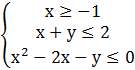### Sample Problem

Solve#### SolutionThe area highlighted in light blue is the area where all of these areas intersect. x≥-1 is the entire area to the right of the line x=-1. x+y≤2 is the entire area under the line y=x-2. x2-2x-y≤0 is the entire area inside the parabola y=(x-1)2-1, therefore the third graph is the one that shows the accurate solution set.

Note: the vertices of the region are (-1, 3) and (2, 0).